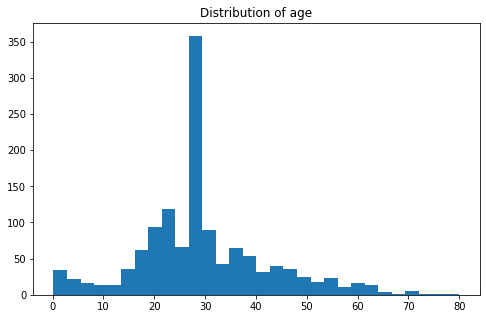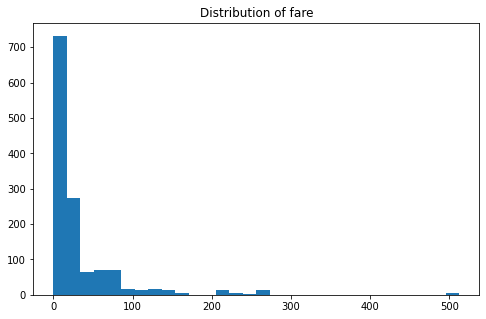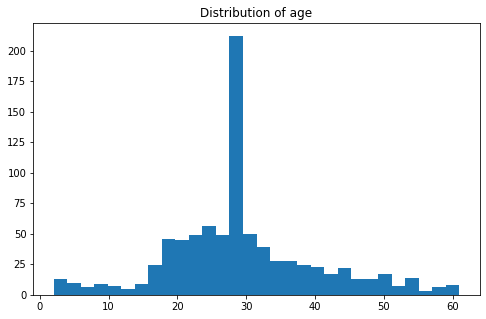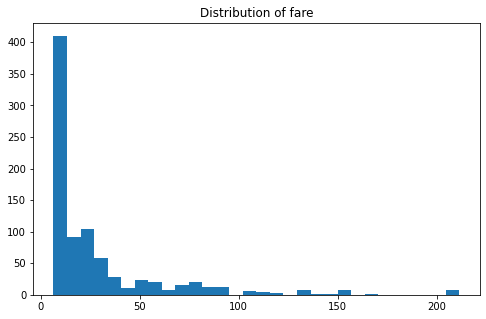# OutlierTrimmer¶

The OutlierTrimmer() removes observations with outliers from the dataset.

It works only with numerical variables. A list of variables can be indicated. Alternatively, the OutlierTrimmer() will select all numerical variables.

The OutlierTrimmer() first calculates the maximum and /or minimum values beyond which a value will be considered an outlier, and thus removed.

Limits are determined using:

• a Gaussian approximation
• the inter-quantile range proximity rule
• percentiles.

### Example:¶

In :
# importing libraries
import pandas as pd
import numpy as np
import matplotlib.pyplot as plt

from sklearn.model_selection import train_test_split

from feature_engine.outliers import OutlierTrimmer

In :
# Load titanic dataset from OpenML

'https://www.openml.org/data/get_csv/16826755/phpMYEkMl')
data = data.replace('?', np.nan)
data['cabin'] = data['cabin'].astype(str).str
data['pclass'] = data['pclass'].astype('O')
data['embarked'].fillna('C', inplace=True)
data['fare'] = data['fare'].astype('float')
data['fare'].fillna(data['fare'].median(), inplace=True)
data['age'] = data['age'].astype('float')
data['age'].fillna(data['age'].median(), inplace=True)
data.drop(['name', 'ticket'], axis=1, inplace=True)
return data

# To plot histogram of given numerical feature

def plot_hist(data, col):
plt.figure(figsize=(8, 5))
plt.hist(data[col], bins=30)
plt.title("Distribution of " + col)
return plt.show()

In :
# Loading titanic dataset
data.sample(5)

Out:
pclass survived sex age sibsp parch fare cabin embarked boat body home.dest
675 3 0 male 21.0 0 0 7.775 n S NaN NaN Brennes, Norway New York
558 2 1 female 18.0 0 2 13.000 n S 16 NaN Finland / Minneapolis, MN
194 1 0 male 30.0 0 0 26.000 C S NaN NaN Brockton, MA
217 1 0 male 64.0 0 0 26.000 n S NaN 263 Isle of Wight, England
473 2 0 male 28.0 0 0 0.000 n S NaN NaN Belfast
In :
# let's separate into training and testing set

X_train, X_test, y_train, y_test = train_test_split(data.drop('survived', axis=1),
data['survived'],
test_size=0.3,
random_state=0)

print("train data shape before removing outliers:", X_train.shape)
print("test data shape before removing outliers:", X_test.shape)

train data shape before removing outliers: (916, 11)
test data shape before removing outliers: (393, 11)

In :
# let's find out the maximum Age and maximum Fare in the titanic

print("Max age:", data.age.max())
print("Max fare:", data.fare.max())

print("Min age:", data.age.min())
print("Min fare:", data.fare.min())

Max age: 80.0
Max fare: 512.3292
Min age: 0.1667
Min fare: 0.0

In :
# Histogram of age feature before capping outliers
plot_hist(data, 'age')In :
# Histogram of fare feature before capping outliers
plot_hist(data, 'fare')### Outlier trimming using Gaussian limits:¶

The transformer will find the maximum and / or minimum values to trim the variables using the Gaussian approximation.

• right tail: mean + 3* std
• left tail: mean - 3* std
In :
'''Parameters
----------

capping_method : str, default=gaussian
Desired capping method. Can take 'gaussian', 'iqr' or 'quantiles'.

tail : str, default=right
Whether to cap outliers on the right, left or both tails of the distribution.
Can take 'left', 'right' or 'both'.

fold: int or float, default=3
How far out to to place the capping values. The number that will multiply
the std or IQR to calculate the capping values.

variables : list, default=None

missing_values: string, default='raise'
Indicates if missing values should be ignored or raised.'''

# removing outliers based on right tail of age and fare columns using gaussian capping method
trimmer = OutlierTrimmer(
capping_method='gaussian', tail='right', fold=3, variables=['age', 'fare'])

# fitting trimmer object to training data
trimmer.fit(X_train)

Out:
OutlierTrimmer(variables=['age', 'fare'])
In :
# here we can find the maximum caps allowed
trimmer.right_tail_caps_

Out:
{'age': 67.49048447470315, 'fare': 174.78162171790441}
In :
# this dictionary is empty, because we selected only right tail
trimmer.left_tail_caps_

Out:
{}
In :
# transforming the training and testing data
train_t = trimmer.transform(X_train)
test_t = trimmer.transform(X_test)

# let's check the new maximum Age and maximum Fare in the titanic
print("Max age:", train_t.age.max())
print("Max fare:", train_t.fare.max())

Max age: 66.0
Max fare: 164.8667

In :
print("train data shape after removing outliers:", train_t.shape)
print(f"{X_train.shape - train_t.shape} observations are removed\n")

print("test data shape after removing outliers:", test_t.shape)
print(f"{X_test.shape - test_t.shape} observations are removed")

train data shape after removing outliers: (887, 11)
29 observations are removed

test data shape after removing outliers: (376, 11)
17 observations are removed


### Gaussian approximation trimming, both tails¶

In :
# Trimming the outliers at both tails using gaussian  method
trimmer = OutlierTrimmer(
capping_method='gaussian', tail='both', fold=2, variables=['fare', 'age'])
trimmer.fit(X_train)

Out:
OutlierTrimmer(fold=2, tail='both', variables=['fare', 'age'])
In :
print("Minimum caps :", trimmer.left_tail_caps_)

print("Maximum caps :", trimmer.right_tail_caps_)

Minimum caps : {'fare': -62.30099726608475, 'age': 4.681562024142586}
Maximum caps : {'fare': 127.36509792110658, 'age': 54.92869998459104}

In :
# transforming the training and testing data
train_t = trimmer.transform(X_train)
test_t = trimmer.transform(X_test)

print("train data shape after removing outliers:", train_t.shape)
print(f"{X_train.shape - train_t.shape} observations are removed\n")

print("test data shape after removing outliers:", test_t.shape)
print(f"{X_test.shape - test_t.shape} observations are removed")

train data shape after removing outliers: (803, 11)
113 observations are removed

test data shape after removing outliers: (334, 11)
59 observations are removed


### Inter Quartile Range, both tails¶

The transformer will find the boundaries using the IQR proximity rule. IQR limits:

• right tail: 75th quantile + 3* IQR
• left tail: 25th quantile - 3* IQR

where IQR is the inter-quartile range: 75th quantile - 25th quantile.

In :
# trimming at both tails using iqr capping method
trimmer = OutlierTrimmer(
capping_method='iqr', tail='both', variables=['age', 'fare'])

trimmer.fit(X_train)

Out:
OutlierTrimmer(capping_method='iqr', tail='both', variables=['age', 'fare'])
In :
print("Minimum caps :", trimmer.left_tail_caps_)

print("Maximum caps :", trimmer.right_tail_caps_)

Minimum caps : {'age': -13.0, 'fare': -62.24179999999999}
Maximum caps : {'age': 71.0, 'fare': 101.4126}

In :
# transforming the training and testing data
train_t = trimmer.transform(X_train)
test_t = trimmer.transform(X_test)

print("train data shape after removing outliers:", train_t.shape)
print(f"{X_train.shape - train_t.shape} observations are removed\n")

print("test data shape after removing outliers:", test_t.shape)
print(f"{X_test.shape - test_t.shape} observations are removed")

train data shape after removing outliers: (857, 11)
59 observations are removed

test data shape after removing outliers: (365, 11)
28 observations are removed


### percentiles or quantiles:¶

The limits are given by the percentiles.

• right tail: 98th percentile
• left tail: 2nd percentile
In :
# trimming at both tails using quantiles capping method
trimmer = OutlierTrimmer(capping_method='quantiles',
tail='both', fold=0.02, variables=['age', 'fare'])

trimmer.fit(X_train)

Out:
OutlierTrimmer(capping_method='quantiles', fold=0.02, tail='both',
variables=['age', 'fare'])
In :
print("Minimum caps :", trimmer.left_tail_caps_)

print("Maximum caps :", trimmer.right_tail_caps_)

Minimum caps : {'age': 2.0, 'fare': 6.44125}
Maximum caps : {'age': 61.69999999999993, 'fare': 211.5}

In :
# transforming the training and testing data
train_t = trimmer.transform(X_train)
test_t = trimmer.transform(X_test)

print("train data shape after removing outliers:", train_t.shape)
print(f"{X_train.shape - train_t.shape} observations are removed\n")

print("test data shape after removing outliers:", test_t.shape)
print(f"{X_test.shape - test_t.shape} observations are removed")

train data shape after removing outliers: (852, 11)
64 observations are removed

test data shape after removing outliers: (358, 11)
35 observations are removed

In :
# Histogram of age feature after removing outliers
plot_hist(train_t, 'age')In :
# Histogram of fare feature after removing outliers
plot_hist(train_t, 'fare')In [ ]: